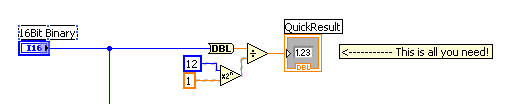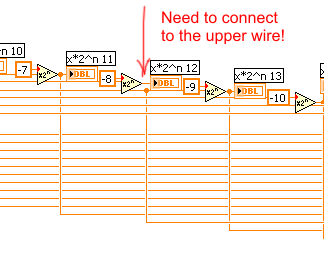# LabVIEW

cancel
Showing results for
Did you mean:

## Converting 16 Bit Integer to Individual Bits

I have a 16 bit Binary Integer and I need to convert this into Individual bits so we can perform some data manipulation.  I know there is a easy solution, but I just cant figure it out.  I attached what I was trying to do, remove the 16 bits and make a 1D array with 16 elements, then remove each element from the array.  This may be the wrong approach to this problem.  Any help would be appreciated.  Thanks in advance.
Message 1 of 10
(3,303 Views)

## Re: Converting 16 Bit Integer to Individual Bits

Forgot to attach.
Message 2 of 10
(3,295 Views)

## Re: Converting 16 Bit Integer to Individual Bits

Hmmm.. at first I wondered: what is a 16-bit binary integer? Never heard of that one. Oh, it's a control set to display in binary mode. Ah....

I'm not sure I completely understand your end goal. Do you want an array of bits? If so, just use the "Number to Boolean Array" function. Do you need this to be an array of ones and zeros? Then wire the output of the "Number to Boolean Array" to the "Boolean to (1,0)" function.
Message 3 of 10
(3,274 Views)

## Re: Converting 16 Bit Integer to Individual Bits

Yep thats what I wanted.  Take the 16bits convert them to boolean array, then boolean to (0,1) array.  Then I could seperate out each one in the array.  Thanks for your help.
Message 4 of 10
(3,262 Views)

## Re: Converting 16 Bit Integer to Individual Bits

If you just need to manipulate a few bits, it is relatively expensive to go the detour via boolean arrays and integer arrays. 😉

Most of the time you can get the desired operation directly via boolean operations on the original number (masking, combining, etc.).

(For a comparison, have a look a the results of the Bit twiddling challenge from a few years ago. Solutions involving a detour via boolean arrays took tens of seconds while direct bit operations did the same tasks in under 100ms. If this is a core part of your code that needs to run in an inner loop with lots of data, optimization with be important.)

What do you actually need to do overall? I am pretty sure there is a better way. Looking at your code, it seems you just want to scale your integer into a fixed point implementation. Most likely, all you need is to divide your input by an integer power of two. 🙂

Keep it simple! (The code you posted is incredibly convoluted!)

Message Edited by altenbach on 05-09-2007 09:33 AMLabVIEW Champion.Message 5 of 10
(3,255 Views)

## Re: Converting 16 Bit Integer to Individual Bits

Yeah, well I couldn't quite figure our what he (or she) wanted either, so I just went by the statement that he wanted individual bits and suggested those two functions to get the array of individual bits. I agree that if the end goal is something else, then a better solution would likely not rely on the use of arrays, and I was not implying by my response that what I suggested was the only (or best) method.

Message Edited by smercurio_fc on 05-09-2007 11:50 AM

Message 6 of 10
(3,249 Views)

## Re: Converting 16 Bit Integer to Individual Bits

It seems your input is a I16 integer, so it is really bad to have the representation of the control as U32. Makes no sense.

Anyway, here's a quick alternative that seems to give the desired result with a code size of less than a postage stamp. see if it works for you. 🙂(note that we get different results for some input values. Do you have the original documentation for the conversion?)

Message Edited by altenbach on 05-09-2007 10:25 AMLabVIEW Champion.Message 7 of 10
(3,244 Views)

## Re: Converting 16 Bit Integer to Individual Bits

I am getting a 16.12 bit signed binary number.  The first bit is multiplied by -2^3, the second bit is multiplied by 2^2, third bit by 2^1, fourth bit by 2^0 then the rest are of bits are multiplied by 2^-1, 2^-2, 2^-3... and so on until 2^-12 to convert them to decimal.  Then all of these are to be added up.  That is what I am trying to do.  I am sure there is a better way to do this.
Message 8 of 10
(3,237 Views)

## Re: Converting 16 Bit Integer to Individual Bits

@TMDATA wrote:
I am getting a 16.12 bit signed binary number.  The first bit is multiplied by -2^3, the second bit is multiplied by 2^2, third bit by 2^1, fourth bit by 2^0 then the rest are of bits are multiplied by 2^-1, 2^-2, 2^-3... and so on until 2^-12 to convert them to decimal.  Then all of these are to be added up.  That is what I am trying to do.  I am sure there is a better way to do this.

OK, that's exacty what my code posted above does! Simple enough? 🙂(Even simpler, you can fold the power of two calculation into a diagram constant.)

The results are not always the same because you have a wiring mistake as shown in the picture.Message Edited by altenbach on 05-09-2007 11:41 AMLabVIEW Champion.Message 9 of 10
(3,225 Views)

## Re: Converting 16 Bit Integer to Individual Bits

Yeah I caught that this morning that the wire was on the wrong one.  I guess thats even more of a reason to make the code simple!  Thanks for the time and effort.
Message 10 of 10
(3,214 Views)